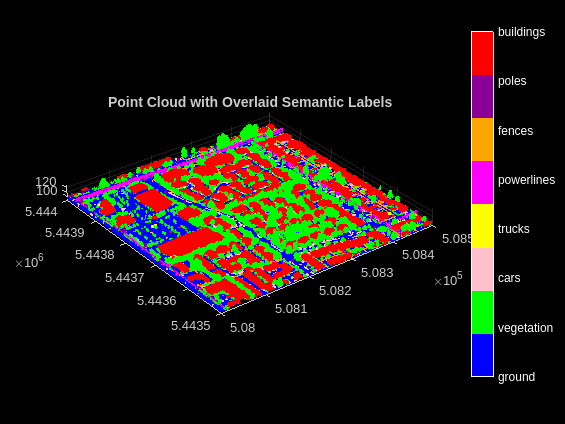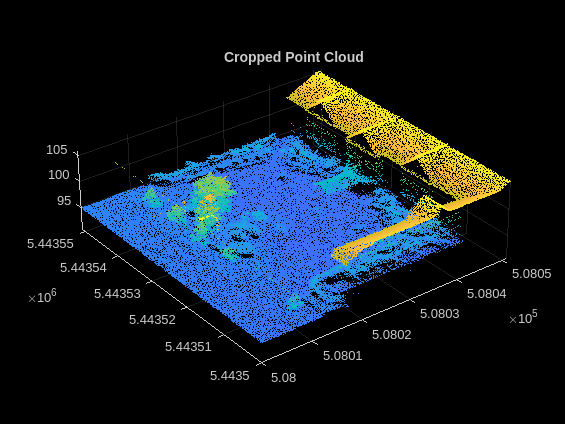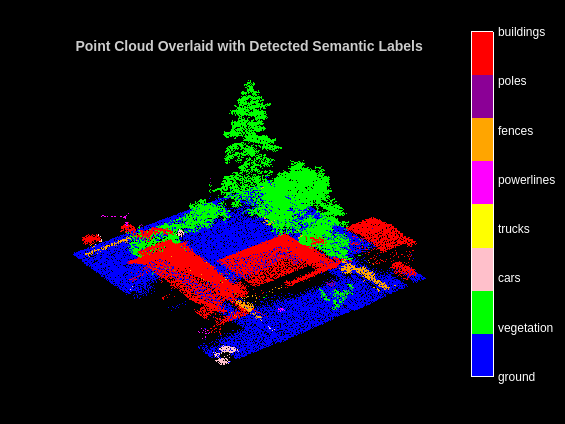# Aerial Lidar Semantic Segmentation Using PointNet++ Deep Learning

This example shows how to train a PointNet++ deep learning network to perform semantic segmentation on aerial lidar data.

Lidar data acquired from airborne laser scanning systems is used in applications such as topographic mapping, city modeling, biomass measurement, and disaster management. Extracting meaningful information from this data requires semantic segmentation, a process where each point in the point cloud is assigned a unique class label.

In this example, you train a PointNet++ network to perform semantic segmentation by using the Dayton Annotated Lidar Earth Scan (DALES) dataset . The dataset contains scenes of dense, labeled aerial lidar data from urban, suburban, rural, and commercial settings. The dataset provides semantic segmentation labels for 8 classes such as buildings, cars, trucks, poles, power lines, fences, ground, and vegetation.

The DALES dataset contains 40 scenes of aerial lidar data. Out of the 40 scenes, 29 scenes are used for training and the remaining 11 scenes are used for testing. Each pixel in the data has a class label. Follow the instructions on the DALES website to download the dataset to the folder specified by the `dataFolder` variable. Create folders to store training and test data.

```dataFolder = fullfile(tempdir,'DALES'); trainDataFolder = fullfile(dataFolder,'dales_las','train'); testDataFolder = fullfile(dataFolder,'dales_las','test');```

Preview a point cloud from the training data.

```lasReader = lasFileReader(fullfile(trainDataFolder,'5080_54435.las')); [pc,attr] = readPointCloud(lasReader,'Attributes','Classification'); labels = attr.Classification; % Select only labeled data. pc = select(pc,labels~=0); labels = labels(labels~=0); classNames = [ "ground" "vegetation" "cars" "trucks" "powerlines" "fences" "poles" "buildings" ]; figure; ax = pcshow(pc.Location,labels); helperLabelColorbar(ax,classNames); title('Point Cloud with Overlaid Semantic Labels');```### Preprocess Data

Each point cloud in the DALES dataset covers an area of 500-by-500 meters, which is much larger than the typical area covered by terrestrial rotating lidar point clouds. For efficient memory processing, divide the point cloud into small, non-overlapping grids.

Use the `helperCropPointCloudsAndMergeLabels` function, attached to this example as a supporting file, to:

• Crop the point clouds into non-overlapping grids of size 50-by-50 meters.

• Downsample the point cloud to a fixed size.

• Normalize the point clouds to range [0 1].

• Save the cropped grids and semantic labels as PCD and PNG files, respectively.

Define the grid dimensions and set a fixed number of points per grid to enable faster training.

```gridSize = [50,50]; numPoints = 8192;```

If the training data is already divided into grids, set `writeFiles` to `false`. Please note that the training data must be in a format supported by the `pcread` function.

```writeFiles = true; numClasses = numel(classNames); [pcCropTrainPath,labelsCropTrainPath,weights] = helperCropPointCloudsAndMergeLabels( ... gridSize,trainDataFolder,numPoints,writeFiles,numClasses);```

Note: Processing can take some time. The code suspends MATLAB® execution until processing is complete.

The point distribution in the training dataset across all the classes is captured in `weights`. Normalize the `weights` using the `maxWeight`.

```[maxWeight,maxLabel] = max(weights); weights = sqrt(maxWeight./weights);```

### Create Datastore Objects for Training

Create a `fileDatastore` object to load PCD files using the `pcread` function.

`ldsTrain = fileDatastore(pcCropTrainPath,'ReadFcn',@(x) pcread(x));`

Use a `pixelLabelDatastore` object to store pixel-wise labels from the pixel label images. The object maps each pixel label to a class name and assigns a unique label ID to each class.

```% Specify label IDs from 1 to the number of classes. labelIDs = 1 : numClasses; pxdsTrain = pixelLabelDatastore(labelsCropTrainPath,classNames,labelIDs);```

Load and display the point cloud.

```ptcld = preview(ldsTrain); labels = preview(pxdsTrain); figure; ax = pcshow(ptcld.Location,uint8(labels)); helperLabelColorbar(ax,classNames); title('Cropped Point Cloud with Overlaid Semantic Labels');```Use the `helperConvertPointCloud` function, defined at the end of this example, to convert the point cloud to cell array. This function also permutes the dimensions of the point cloud to make it compatible with the input layer of the network.

`ldsTransformed = transform(ldsTrain,@(x) helperConvertPointCloud(x));`

Use the `combine` function to combine the point clouds and pixel labels into a single datastore for training.

`dsTrain = combine(ldsTransformed,pxdsTrain);`

### Define PointNet++ Model

The PointNet++  segmentation model consists of two main components:

• Set abstraction modules

• Feature propagation modules

The series of set abstraction modules progressively subsamples points of interest by hierarchically grouping points, and uses a custom PointNet architecture to encode points into feature vectors. Because semantic segmentation tasks require point features for all the original points, a series of feature propagation modules are used to hierarchically interpolate features to original points using an inverse-distance based interpolation scheme.

Define the PointNet++ architecture using the `pointnetplusLayers` function.

`lgraph = pointnetplusLayers(numPoints,3,numClasses);`

To handle the class-imbalance on the DALES dataset, the weighted cross-entropy loss from the `pixelClassificationLayer` function is used. This will penalize the network more if a point belonging to a class with lower weight is misclassified. ` `

```% Replace the FocalLoss layer with pixelClassificationLayer. larray = pixelClassificationLayer('Name','SegmentationLayer','ClassWeights', ... weights,'Classes',classNames); lgraph = replaceLayer(lgraph,'FocalLoss',larray);```

### Specify Training Options

Use the A`dam` optimization algorithm to train the network. Use the `trainingOptions` function to specify the hyperparameters.

```learningRate = 0.0005; l2Regularization = 0.01; numEpochs = 20; miniBatchSize = 6; learnRateDropFactor = 0.1; learnRateDropPeriod = 10; gradientDecayFactor = 0.9; squaredGradientDecayFactor = 0.999; options = trainingOptions('adam', ... 'InitialLearnRate',learningRate, ... 'L2Regularization',l2Regularization, ... 'MaxEpochs',numEpochs, ... 'MiniBatchSize',miniBatchSize, ... 'LearnRateSchedule','piecewise', ... 'LearnRateDropFactor',learnRateDropFactor, ... 'LearnRateDropPeriod',learnRateDropPeriod, ... 'GradientDecayFactor',gradientDecayFactor, ... 'SquaredGradientDecayFactor',squaredGradientDecayFactor, ... 'Plots','training-progress');```

Note: Reduce the `miniBatchSize` value to control memory usage when training.

### Train Model

You can train the network yourself by setting the `doTraining` argument to `true`. If you train the network, you can use a CPU or GPU. Using a GPU requires Parallel Computing Toolbox™ and a CUDA® enabled NVIDIA® GPU. For more information, see GPU Support by Release (Parallel Computing Toolbox). Otherwise, load a pretrained network.

```doTraining = false; if doTraining % Train the network on the dsTrain datastore using the trainNetwork function. [net, info] = trainNetwork(dsTrain,lgraph,options); else % Load the pretrained network. load('pointnetplusTrained.mat','net'); end```

### Segment Aerial Point Cloud

The network is trained on downsampled point clouds. To perform segmentation on a test point cloud, first downsample the test point cloud, similar to how training data is downsampled. Perform inference on this downsampled test point cloud to compute prediction labels. Interpolate the prediction labels, to obtain prediction labels on the dense point cloud.

Define `numNearestNeighbors` and `radius` to find the nearest points in the downsampled point cloud for each point in the dense point cloud and to perform interpolation effectively.

```numNearestNeighbors = 20; radius = 0.05;```

Read the full test point cloud.

```lasReader = lasFileReader(fullfile(testDataFolder,'5080_54470.las')); [pc,attr] = readPointCloud(lasReader,'Attributes','Classification'); labelsDenseTarget = attr.Classification; % Select only labeled data. pc = select(pc,labelsDenseTarget~=0); labelsDenseTarget = labelsDenseTarget(labelsDenseTarget~=0); % Initialize prediction labels labelsDensePred = zeros(size(labelsDenseTarget));```

Calculate the number of non-overlapping grids based on `gridSize`, `XLimits`, and `YLimits` of the point cloud.

```numGridsX = round(diff(pc.XLimits)/gridSize(1)); numGridsY = round(diff(pc.YLimits)/gridSize(2)); [~,edgesX,edgesY,indx,indy] = histcounts2(pc.Location(:,1),pc.Location(:,2), ... [numGridsX,numGridsY],'XBinLimits',pc.XLimits,'YBinLimits',pc.YLimits); ind = sub2ind([numGridsX,numGridsY],indx,indy);```

Iterate over all the non-overlapping grids and predict the labels using the `semanticseg` `f`unction.

```for num=1:numGridsX*numGridsY idx = ind==num; ptCloudDense = select(pc,idx); labelsDense = labelsDenseTarget(idx); % Use the helperDownsamplePoints function, attached to this example as a % supporting file, to extract a downsampled point cloud from the % dense point cloud. ptCloudSparse = helperDownsamplePoints(ptCloudDense, ... labelsDense,numPoints); % Make the spatial extent of the dense point cloud and the sparse point % cloud same. limits = [ptCloudDense.XLimits;ptCloudDense.YLimits;ptCloudDense.ZLimits]; ptCloudSparseLocation = ptCloudSparse.Location; ptCloudSparseLocation(1:2,:) = limits(:,1:2)'; ptCloudSparse = pointCloud(ptCloudSparseLocation,'Color',ptCloudSparse.Color, ... 'Intensity',ptCloudSparse.Intensity, ... 'Normal',ptCloudSparse.Normal); % Use the helperNormalizePointCloud function, attached to this example as % a supporting file, to normalize the point cloud between 0 and 1. ptCloudSparseNormalized = helperNormalizePointCloud(ptCloudSparse); ptCloudDenseNormalized = helperNormalizePointCloud(ptCloudDense); % Use the helperConvertPointCloud function, defined at the end of this % example, to convert the point cloud to a cell array and to permute the % dimensions of the point cloud to make it compatible with the input layer % of the network. ptCloudSparseForPrediction = helperConvertPointCloud(ptCloudSparseNormalized); % Get the output predictions. labelsSparsePred = semanticseg(ptCloudSparseForPrediction{1,1}, ... net,'OutputType','uint8'); % Use the helperInterpolate function, attached to this example as a % supporting file, to calculate labels for the dense point cloud, % using the sparse point cloud and labels predicted on the sparse point cloud. interpolatedLabels = helperInterpolate(ptCloudDenseNormalized, ... ptCloudSparseNormalized,labelsSparsePred,numNearestNeighbors, ... radius,maxLabel,numClasses); labelsDensePred(idx) = interpolatedLabels; end```
```Starting parallel pool (parpool) using the 'local' profile ... Connected to the parallel pool (number of workers: 6). ```

For better visualisation, select a region of interest from the point cloud data. Modify the limits in the `roi` variable according to the point cloud data.

```roi = [edgesX(5) edgesX(8) edgesY(8) edgesY(11) pc.ZLimits]; indices = findPointsInROI(pc,roi); figure; ax = pcshow(select(pc,indices).Location, labelsDensePred(indices)); axis off; zoom(ax,1.5); helperLabelColorbar(ax,classNames); title('Point Cloud Overlaid with Detected Semantic Labels');```### Evaluate Network

Evaluate the network performance on the test data. Use the `evaluateSemanticSegmentation` function to compute the semantic segmentation metrics from the test set results. The target and predicted labels are computed previously and are stored in the `labelsDensePred` and the `labelsDenseTarget` variables respectively.

```confusionMatrix = segmentationConfusionMatrix(labelsDensePred, ... double(labelsDenseTarget),'Classes',1:numClasses); metrics = evaluateSemanticSegmentation({confusionMatrix},classNames,'Verbose',false);```

You can measure the amount of overlap per class using the intersection-over-union (IoU) metric.

The `evaluateSemanticSegmentation` function returns metrics for the entire data set, for individual classes, and for each test image. To see the metrics at the data set level, use the `metrics.DataSetMetrics` property.

`metrics.DataSetMetrics`
```ans=1×4 table GlobalAccuracy MeanAccuracy MeanIoU WeightedIoU ______________ ____________ _______ ___________ 0.93191 0.64238 0.52709 0.88198 ```

The data set metrics provide a high-level overview of network performance. To see the impact each class has on the overall performance, inspect the metrics for each class using the `metrics.ClassMetrics` property.

`metrics.ClassMetrics`
```ans=8×2 table Accuracy IoU ________ _________ ground 0.98874 0.93499 vegetation 0.85948 0.81865 cars 0.61847 0.36659 trucks 0.018676 0.0070006 powerlines 0.7758 0.6904 fences 0.3753 0.21718 poles 0.5741 0.28528 buildings 0.92843 0.89662 ```

Although the overall network performance is good, the class metrics for some classes like `Trucks` indicate that more training data is required for better performance.

### Supporting Functions

The `helperLabelColorbar` function adds a colorbar to the current axis. The colorbar is formatted to display the class names with the color.

```function helperLabelColorbar(ax,classNames) % Colormap for the original classes. cmap = [[0,0,255]; [0,255,0]; [255,192,203]; [255,255,0]; [255,0,255]; [255,165,0]; [139,0,150]; [255,0,0]]; cmap = cmap./255; cmap = cmap(1:numel(classNames),:); colormap(ax,cmap); % Add colorbar to current figure. c = colorbar(ax); c.Color = 'w'; % Center tick labels and use class names for tick marks. numClasses = size(classNames, 1); c.Ticks = 1:1:numClasses; c.TickLabels = classNames; % Remove tick mark. c.TickLength = 0; end```

The `helperConvertPointCloud` function converts the point cloud to a cell array and permutes the dimensions of the point cloud to make it compatible with the input layer of the network.

```function data = helperConvertPointCloud(data) if ~iscell(data) data = {data}; end numObservations = size(data,1); for i = 1:numObservations tmp = data{i,1}.Location; data{i,1} = permute(tmp,[1,3,2]); end end```

### References

 Varney, Nina, Vijayan K. Asari, and Quinn Graehling. "DALES: A Large-Scale Aerial LiDAR dataset for Semantic Segmentation." ArXiv:2004.11985 [Cs, Stat], April 14, 2020. https://arxiv.org/abs/2004.11985.

 Qi, Charles R., Li Yi, Hao Su, and Leonidas J. Guibas. "PointNet++: Deep Hierarchical Feature Learning on Point Sets in a Metric Space." ArXiv:1706.02413 [Cs], June 7, 2017. https://arxiv.org/abs/1706.02413.

## Support